# Negative frequencies

I recently asked for your advice about how to continue my Fourier transform series. I appreciate all the enthusiastic and helpful responses. I'd like to continue focusing on some basic points that confuse folks who don't have an extensive signal processing background:

• Negative frequencies
• Use of fftshift
• Complex-valued outputs from fft function
• DTFT plotting procedure
• Physical units on DTFT plots
• Revisit two-dimensional frequency domain visualization for images
• Significance of frequency-domain phase for images
• Two-image magnitude-phase swapping experiment

I will leave the more advanced signal processing topics for a later time.

Now, onto negative frequencies. Here are the plots of a cosine signal and its continuous-time Fourier transform (reproduced from my December 11, 2009 post.)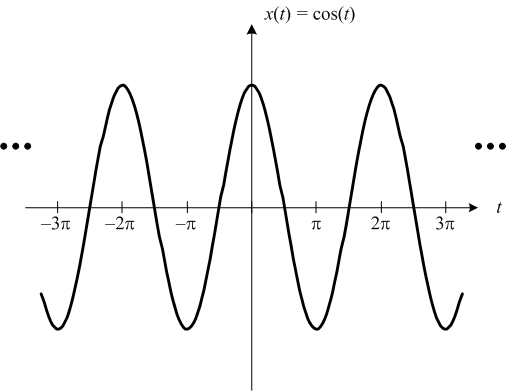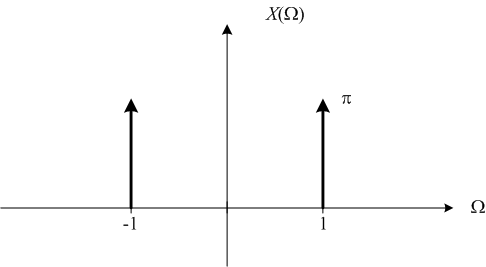What's that impulse at -1 all about, anyway?

Imagine that you gathered 10 people, all knowledgeable about signal processing and Fourier transforms, into a room and asked them to explain about negative frequencies. It is a well-established fact that you will get 27.4 different explanations. And three of the experts will get into a shouting match over which explanations are correct. (This experiment can be dangerous; please don't try it at home.)

So it is with some trepidation that I offer you a couple of different ways to think about negative frequencies.

1. Let's start with a simple cosine signal,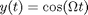. The frequency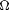can be positive or negative (e.g.,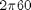or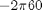). This is a useful distinction to make. (Is that wheel thingy turning forward or backward?)

2. The Fourier transform uses a kind of complex exponential signal for its basis functions, not real-valued sinusoids. The complex exponential used is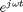, where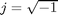(as all electrical engineers know), real-valuedis the frequency in radians per second, andis time. This function is related to the real-valued cosine and sine functions as follows: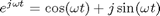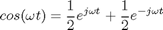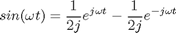So you can see that when you express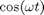in terms of complex exponentials, there is both a positive frequency and a negative frequency term.

3. Finally, if you think the whole idea of complex-valued time-domain signals is just weird, they are actually quite useful in many domains. In the area of communications, for example, some systems transmit information as two different signals (the in-phase component and the quadrature-phase component). It is very convenient to model these signals mathematically as the real and imaginary parts of a single complex-valued signal. These complex-valued signals might have only positive (or only negative) frequency components.

Do you have your own favorite way to explain negative frequencies? Please share it with us here by posting a comment.

Published with MATLAB® 7.10

|